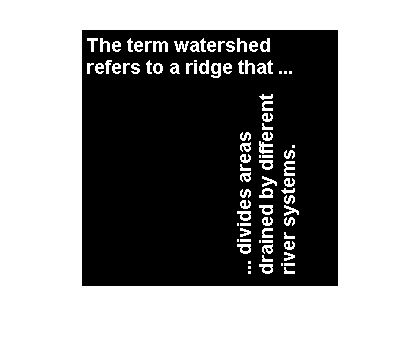# Packed dilation and erosion

This post is another in my series on morphological dilation and erosion algorithms.

One of the algorithm techniques used by imdilate and imerode is binary image bit packing. In bit packing, groups of 32 binary image pixels are stored as bits in unsigned 32-bit integers.

The Image Processing Toolbox functions bwpack and bwunpack do the packing and unpacking. Let's look at a simple example, starting with reading in text.png and then extracting a 32-by-4 subimage.

bw = imread('text.png');
imshow(bw)bw_sub = bw(12:41, 30:33)
bw_sub =

0     0     0     0
0     0     1     1
0     0     1     1
0     1     1     1
0     1     1     1
0     1     1     1
0     1     1     1
0     1     1     1
0     0     1     1
0     0     1     1
0     0     0     0
0     0     0     0
0     0     0     0
0     0     0     0
0     0     0     0
0     0     0     0
0     0     0     0
0     0     0     0
0     0     0     0
1     0     0     0
1     0     0     0
0     0     0     0
0     0     0     0
0     0     1     1
0     0     1     1
0     1     1     1
0     1     1     1
0     1     1     1
0     1     1     1
0     1     1     1



Now pass the subimage to bwpack.

bw_sub_packed = bwpack(bw_sub)
whos
bw_sub_packed =

1572864  1040187640  1065354238  1065354238

Name                 Size             Bytes  Class      Attributes

bw                 256x256            65536  logical
bw_sub              30x4                120  logical
bw_sub_packed        1x4                 16  uint32



bw_sub_packed is just a 1-by-4 uint32 matrix, but those 4 values contain all the values in the original 32-by-4 subimage. You can see the correspondence by using the dec2bin function, which converts a decimal number to binary form (represented as a string).

% Convert the bw_sub_packed(1,2) to binary form.
dec2bin(bw_sub_packed(1, 2))
ans =

111110000000000000000011111000



You can see that these are the 0 and 1 values from the second column of bw_sub.

So what does this have to do with dilation and erosion? Binary image dilation and erosion can be expressed in terms of translations, logical ANDs, and logical ORs of pixel values. The C and C++ operators >>, <<, &, and | operate on unsigned integer values in bitwise fashion. Therefore, by packing binary image pixel values into the bits of unsigned 32-bit integers, we can write C or C++ code that dilates and erodes faster, because it is essentially operating on multiple pixel values at a time.

Let's try dilating text.png by packing it, passing the packed image to imdilate, and then unpacking the result. (Note that imdilate supports 32-bit integer images, so we have to explicitly tell imdilate to treat the input as packed.) We'll use a diagonal cross structuring element.

bw_packed = bwpack(bw);
se = strel(eye(9) | rot90(eye(9)));
bw_packed_dilated = imdilate(bw_packed, se, 'ispacked');
bw_dilated = bwunpack(bw_packed_dilated);
imshow(bw_dilated)Let's time packed dilation and compare it to the ordinary version, using a 2k-by-2k image.

bw = repmat(bw, 8, 8);
bw_packed = bwpack(bw);
packed_dilation_time = timeit(@() imdilate(bw_packed, se, 'ispacked'))
packed_dilation_time =

0.0127


ordinary_dilation_time = timeit(@() imdilate(bw, se))
ordinary_dilation_time =

0.0396


speedup = ordinary_dilation_time / packed_dilation_time
speedup =

3.1251



The measured speedup is good, but much less than a factor of 32, which we might have expected. There are two reasons for the smaller speedup factor:

• Input argument parsing and other overhead in imdilate.m
• The C++ code used by the toolbox for ordinary dilation uses a method that does better when there are relatively few white input pixels, and this method can't be used as effectively for the packed version.

Here's a design question for you to consider: Should imdilate always use the packed form? In other words, if you pass it a logical input, should imdilate pack it, performed packed dilation, and then unpack it?

Well, the packing and unpacking steps take time. For example:

timeit(@() bwpack(bw))
ans =

0.0198



So it's conceivable that packing, packed dilation, and unpacking could together take longer than the ordinary dilation implementation.

We decided that imdilate (and imerode) should automatically do bit packing only if the structuring element is decomposed into at least two structuring elements. That way, the cost of packing and unpacking is amortized across the cost of at least two packed dilation operations.

Lately, we've been taking a fresh look at various aspects of our morphology operations, and we're optimistic that we can make them go faster in future releases of the Image Processing Toolbox.

Published with MATLAB® 7.7

|